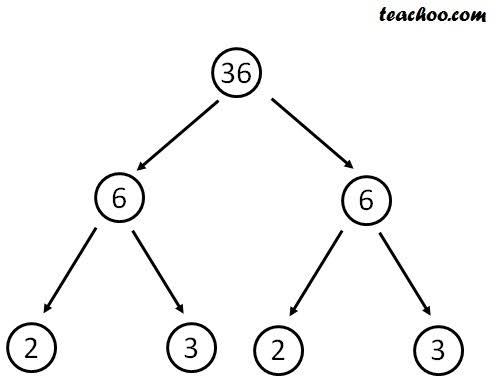# Factors Of 36 | Factors Of 36 In Pairs

Factors are the number that gives you the exact answer when multiplied together means when you multiply them, you will get the number 36. Here we will discuss the factors of 36 and the different methods used to find the factors of 36.

## What Are The Factors Of 36?

Here are all the factors of 36.

Positive Factors: 1, 2, 3, 4, 6, 9, 12, 18, 36

Negative Factors: -1, -2, -3, -4, -6, -9, -12, -18, -36

Prime Factorization: 2 × 2 × 3 ×3 = 22 × 32

Prime Factors: 2, 3

Sum Of Factors: 91

## Factors Of 36 In Pairs

There are two different pair factors of 36, among which one are positive factors while the other are negative factors. Here are both sets of factors;

Positive Factors: ( 1, 36 ), ( 2, 18 ), ( 3, 12 ), ( 4, 9 ), ( 6, 6 )

Negative Factors: (-1, -36 ), ( -2, -18 ), ( -3, -12 ), ( -4, -9 ), ( -6, -6 )

## Factor Tree of 36Pic credit: Teachoo.com

## Step By Step Guide Of 36 Divided By 9

Let’s divide 36 by 9 step by step to make it easier for you to learn about it. You can use this method to divide other numbers too.

Step 1:

In the first step, write the numbers in the correct form using the long division symbol first, and then write the divisor on the left side while the dividend is on the right side. Check this out.

9 ⟌3 6

Step 2:

Now put 0 at the top of the Long division symbol as 9, or the divisor goes to the first digit of dividend 0 times.

0

9  ⟌3 6

Step 3:

Now multiply the divisor with the result and write the answer below the dividend.

First, multiply it,

9 × 0 = 0

Now do this,

0

9 ⟌3 6

0

Step 4:

Now subtract the result from the dividend like 3 - 0 = 3.

0

9 ⟌3 6

-   0

_____

3

Step 5:

Now bring the other number of the dividend down there.

0

9 ⟌3 6

-   0

_____

3 6

Step 6:

Now the number 9 goes 4 times to the number 36. So, write it on the top of the equation.

0 4

9 ⟌3 6

-   0

_____

3 6

Step 7:

Now multiply the divisor with the result to verify your answer ( 9 × 4 = 36 ).

0  4

9 ⟌3 6

-   0

_____

3 6

3 6

Step 8:

Now subtract the result and write it below.

0 4

9 ⟌3 6

-   0

_____

3 6

-  3 6

_____

0

So the answer to your question is 4 as there isn’t any number left with whom you can carry further. While the Remainder of this question is 0.

## Step By Step Guide Of 36 Divided By 4

Step 1:

In the first step, write the numbers in the correct form using the long division symbol first, and then write the divisor on the left side while the dividend is on the right side. Check this out.

4 ⟌3 6

Step 2:

Now put 0 at the top of the Long division symbol as 4, or the divisor goes to the first digit of dividend 0 times.

0

4 ⟌3 6

Step 3:

Now multiply the divisor with the result and write the answer below the dividend.

First, multiply it,

4 × 0 = 0

Now do this,

0

4 ⟌3 6

0

Step 4:

Now subtract the result from the dividend like 3 - 0 = 3.

0

4 ⟌3 6

-   0

_____

3

Step 5:

Now bring the other number of the dividend down there.

0

4 ⟌3 6

-   0

_____

3 6

Step 6:

Now the number 4 goes 9 times to the number 36. So, write it on the top of the equation.

0 9

4 ⟌3 6

-   0

_____

3 6

Step 7:

Now multiply the divisor with the result to verify your answer ( 4 × 9 = 36 ).

0  9

4 ⟌3 6

-   0

_____

3 6

3 6

Step 8:

Now subtract the result and write it below.

0 9

4 ⟌3 6

-   0

_____

3 6

-  3 6

_____

0